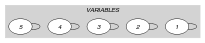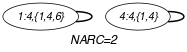## 5.121. discrepancy

Origin
Constraint

$\mathrm{𝚍𝚒𝚜𝚌𝚛𝚎𝚙𝚊𝚗𝚌𝚢}\left(\mathrm{𝚅𝙰𝚁𝙸𝙰𝙱𝙻𝙴𝚂},𝙺\right)$

Arguments
 $\mathrm{𝚅𝙰𝚁𝙸𝙰𝙱𝙻𝙴𝚂}$ $\mathrm{𝚌𝚘𝚕𝚕𝚎𝚌𝚝𝚒𝚘𝚗}\left(\mathrm{𝚟𝚊𝚛}-\mathrm{𝚍𝚟𝚊𝚛},\mathrm{𝚋𝚊𝚍}-\mathrm{𝚜𝚒𝚗𝚝}\right)$ $𝙺$ $\mathrm{𝚒𝚗𝚝}$
Restrictions
 $\mathrm{𝚛𝚎𝚚𝚞𝚒𝚛𝚎𝚍}$$\left(\mathrm{𝚅𝙰𝚁𝙸𝙰𝙱𝙻𝙴𝚂},\mathrm{𝚟𝚊𝚛}\right)$ $\mathrm{𝚛𝚎𝚚𝚞𝚒𝚛𝚎𝚍}$$\left(\mathrm{𝚅𝙰𝚁𝙸𝙰𝙱𝙻𝙴𝚂},\mathrm{𝚋𝚊𝚍}\right)$ $𝙺\ge 0$ $𝙺\le |\mathrm{𝚅𝙰𝚁𝙸𝙰𝙱𝙻𝙴𝚂}|$
Purpose

$𝙺$ is the number of variables of the collection $\mathrm{𝚅𝙰𝚁𝙸𝙰𝙱𝙻𝙴𝚂}$ that take their value in their respective sets of bad values.

Example
$\left(\begin{array}{c}〈\begin{array}{cc}\mathrm{𝚟𝚊𝚛}-4\hfill & \mathrm{𝚋𝚊𝚍}-\left\{1,4,6\right\},\hfill \\ \mathrm{𝚟𝚊𝚛}-5\hfill & \mathrm{𝚋𝚊𝚍}-\left\{0,1\right\},\hfill \\ \mathrm{𝚟𝚊𝚛}-5\hfill & \mathrm{𝚋𝚊𝚍}-\left\{1,6,9\right\},\hfill \\ \mathrm{𝚟𝚊𝚛}-4\hfill & \mathrm{𝚋𝚊𝚍}-\left\{1,4\right\},\hfill \\ \mathrm{𝚟𝚊𝚛}-1\hfill & \mathrm{𝚋𝚊𝚍}-\varnothing \hfill \end{array}〉,2\hfill \end{array}\right)$

The $\mathrm{𝚍𝚒𝚜𝚌𝚛𝚎𝚙𝚊𝚗𝚌𝚢}$ constraint holds since exactly $𝙺=2$ variables (i.e., the first and fourth variables) of the $\mathrm{𝚅𝙰𝚁𝙸𝙰𝙱𝙻𝙴𝚂}$ collection take their value within their respective sets of bad values.

Typical
 $|\mathrm{𝚅𝙰𝚁𝙸𝙰𝙱𝙻𝙴𝚂}|>1$ $𝙺<|\mathrm{𝚅𝙰𝚁𝙸𝙰𝙱𝙻𝙴𝚂}|$
Symmetries
• Items of $\mathrm{𝚅𝙰𝚁𝙸𝙰𝙱𝙻𝙴𝚂}$ are permutable.

• All occurrences of two distinct values in $\mathrm{𝚅𝙰𝚁𝙸𝙰𝙱𝙻𝙴𝚂}.\mathrm{𝚟𝚊𝚛}$ or $\mathrm{𝚅𝙰𝚁𝙸𝙰𝙱𝙻𝙴𝚂}.\mathrm{𝚋𝚊𝚍}$ can be swapped; all occurrences of a value in $\mathrm{𝚅𝙰𝚁𝙸𝙰𝙱𝙻𝙴𝚂}.\mathrm{𝚟𝚊𝚛}$ or $\mathrm{𝚅𝙰𝚁𝙸𝙰𝙱𝙻𝙴𝚂}.\mathrm{𝚋𝚊𝚍}$ can be renamed to any unused value.

Arg. properties
• Functional dependency: $𝙺$ determined by $\mathrm{𝚅𝙰𝚁𝙸𝙰𝙱𝙻𝙴𝚂}$.

• Aggregate: $\mathrm{𝚅𝙰𝚁𝙸𝙰𝙱𝙻𝙴𝚂}\left(\mathrm{𝚞𝚗𝚒𝚘𝚗}\right)$, $𝙺\left(+\right)$.

Remark

Limited discrepancy search was first introduced by M. L. Ginsberg and W. D. Harvey as a search technique in [GinsbergHarvey95]. Later on, discrepancy based filtering was presented in the PhD thesis of F. Focacci [Focacci01]. Finally the $\mathrm{𝚍𝚒𝚜𝚌𝚛𝚎𝚙𝚊𝚗𝚌𝚢}$ constraint was explicitly defined in the PhD thesis of W.-J. van Hoeve [vanHoeve05].

Keywords
Arc input(s)

$\mathrm{𝚅𝙰𝚁𝙸𝙰𝙱𝙻𝙴𝚂}$

Arc generator
$\mathrm{𝑆𝐸𝐿𝐹}$$↦\mathrm{𝚌𝚘𝚕𝚕𝚎𝚌𝚝𝚒𝚘𝚗}\left(\mathrm{𝚟𝚊𝚛𝚒𝚊𝚋𝚕𝚎𝚜}\right)$

Arc arity
Arc constraint(s)
$\mathrm{𝚒𝚗}_\mathrm{𝚜𝚎𝚝}$$\left(\mathrm{𝚟𝚊𝚛𝚒𝚊𝚋𝚕𝚎𝚜}.\mathrm{𝚟𝚊𝚛},\mathrm{𝚟𝚊𝚛𝚒𝚊𝚋𝚕𝚎𝚜}.\mathrm{𝚋𝚊𝚍}\right)$
Graph property(ies)
$\mathrm{𝐍𝐀𝐑𝐂}$$=𝙺$

Graph model

The arc constraint corresponds to the constraint $\mathrm{𝚒𝚗}_\mathrm{𝚜𝚎𝚝}\left(\mathrm{𝚟𝚊𝚛𝚒𝚊𝚋𝚕𝚎𝚜}.\mathrm{𝚟𝚊𝚛},\mathrm{𝚟𝚊𝚛𝚒𝚊𝚋𝚕𝚎𝚜}.\mathrm{𝚋𝚊𝚍}\right)$ defined in this catalogue. We employ the $\mathrm{𝑆𝐸𝐿𝐹}$ arc generator in order to produce an initial graph with a single loop on each vertex.

Parts (A) and (B) of Figure 5.121.1 respectively show the initial and final graph associated with the Example slot. Since we use the $\mathrm{𝐍𝐀𝐑𝐂}$ graph property, the loops of the final graph are stressed in bold.

##### Figure 5.121.1. Initial and final graph of the $\mathrm{𝚍𝚒𝚜𝚌𝚛𝚎𝚙𝚊𝚗𝚌𝚢}$ constraint(a) (b)